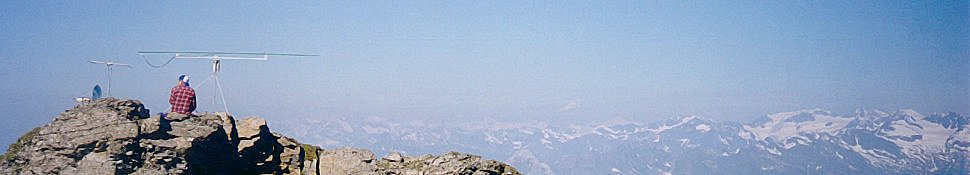# Some ham programs and online tools

Here are some programs and online forms I have developed trying to solve some common design problems.

## Computing the best resistors combinations

Ever wondered which combination of two resistors give you the closest match to that strange value you need for your latest circuit ? This resistors calculator has the answer, computing all the combinations of two resistors (series or parallel) in the E12 (10 %), E24 (5 %) or E96 (1 %) series that gives an approximation better than the closest standard value.

## Computing the inductance of single-layer coils

Here is a form to compute the inductance of a single-layer circular coil with no restriction on the diameter-to-length ratio.

## Computing the inductance of one-turn loops

One-turn loops are often used as receiving (sometimes also transmitting) antennas; here you can compute the inductance of a one-turn loop with different shapes (round, square, triangular, etc.); a similar form for rectangular loops of round and rectangular wire is available, together with a comparison of different formulas and simulation results

## Estimate the self-capacitance of an inductor

A real inductor behaves actually (as most practical components) as a combination of inductance, resistance and capacitance. The parasitic capacitance appears between its turn and can be thought as in parallel with its overall inductance (at least at low frequencies). This makes the inductor self resonant at some frequency and can cause some difficult in measuring the inductance since the capacitive reactance cancels out some of the inductive reactance, making the apparent inductance value bigger than the actual value. Moreover, above resonance, the capacitive effect will prevail and the inductor will behave as a capacitance!.
This online tool helps in determining the real inductance value and gives an estimation of the parasitic capacitance.
As an example, here you can find the inductance and self-capacitance values of my variometer computed using this method.

## Designing a variometer (variable inductor)

A variable inductor (usually called variometer) can be very helpful in tuning antennas, especially at low frequencies, where it is often the main tuner component; at 137 kHz it is usually the only component needed in the matching network!.
The most common type of variometer consists of two coils connected in series that can be rotated with respect to each other in order to vary the mutual inductance; another type has the coils orientation fixed and varies the axial distance between the two. I have prepared a simple form that computes the main parameters for these two kind of variometers.
As a practical example, I designed a variometer for tuning my vertical antenna on 137 kHz, taking the size and the available wire length as main constraints. Some pictures of this variometer can be found in the pictures page.

## Computing the inductance of arbitrary coils configurations

Since calculating the inductance for generic cases via an analytical approach is viable only for simple structures before the math becomes too complex (no pun intended), one has to resort to electromagnetic simulation programs to know the inductance of not-so-common configurations. Simulations are also useful when the standard formulas are not applicable, as with coils with widely spaced turns.
A well-known tool used for computation of the inductance of arbitrary 3D structures is fasthenry, whose source code is available at the MIT Research Laboratory of Electronics Computational Prototyping Group.
I have written a simple program, coilgen to quickly generate fasthenry input files for arbitrary coils systems, to allow to efficiently evaluate the performances of variometers and coils designs.

## Designing narrowband bandpass filters

I have written a simple program to design narrowband bandpass filters; supported filter types are equiripple (Tchebyscheff), maximally flat (Butterworth) and minimum loss (Cohn).

## Receiving and transmitting QRSS and DFCW with Linux

QRSS, also called slow CW is a widely used technique for communications in the LF bands (137 and 73 kHz, where available...); it is much like normal CW, but with a dot length measured in terms of seconds, some time even minutes! DFCW is a related mode, in which the dash and dots have the same duration, but are transmitted using two (slightly) different frequencies. This allows to detect very low level signals by using spectral analysis on a computer.

glfer is a program I wrote in C under Linux using the GTK+ library, supporting reception and transmission of QRSS and DFCW signals. More information are available at the glfer page, where you can also download the source code.
For *indows users there are similar (well, I should say better...) programs supporting these visual modes, for example

• EasyGram by Petr Maly, OK1FIG   (transmission and reception)
• QRS by Rik Strobbe, ON7YD   (transmission only)
These programs (and many others) can be found also at the NL9222 software page.

## Analyzing diode mixers

DIODEMX is a diode mixers analysis program, derived from the original Turbo Pascal sources by Stephen A. Maas, retaining all the original features. It includes noise and intermodulation analysis, conjugate-match calculations and graphical plotting of the results..

## Creating disks for rotary encoders

If you are thinking of building your own antenna positioning system, maybe you will need to draw some disks for the rotary encoders. Here you can find two simple postscript files for printing rotary encoders disks, that are fully configurable (see comments in the first example) for (almost) any size and resolution (i.e. number of bits).